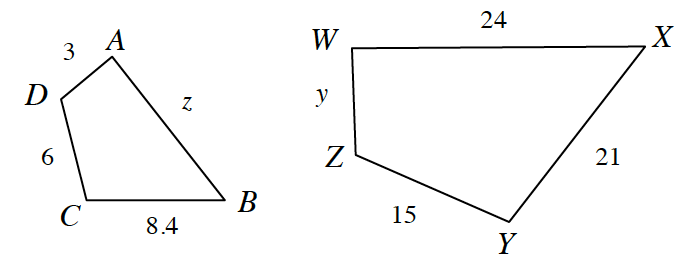### Home > CCG > Chapter 3 > Lesson 3.2.6 > Problem3-113

3-113.

List a sequence of transformations that demonstrates $ABCD\sim WXYZ$, then find $y$ and $z$.What two transformations are needed to move $ABCD$ onto $WXYZ$?

To find $z$ and $y$, divide or multiply by the zoom factor.

$z=9.6$